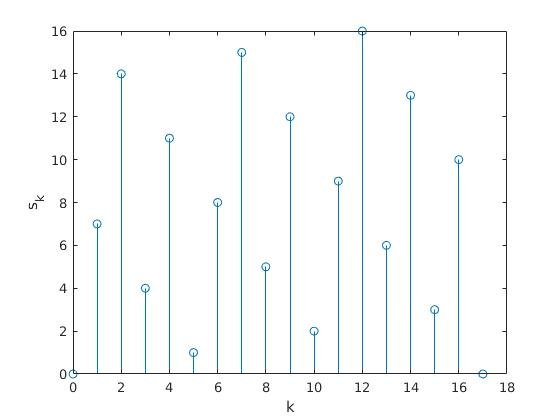Categorías

# Pseudo-random number generator with Fibonacci sequence

$s_k = (k\cdot A) \bmod B$

$$s_k$$ is the pseudo-random number and $$A$$ and $$B$$ are prime numbers. $$k$$ is in the range $$[0,B-1]$$. If $$k$$ is greater than $$B-1$$, the results will be repeat as $$B$$ is the period of the sequence.
For example, $$A = 7$$ and $$B = 17$$. This sequence written in MATLAB could be:

A = 7;
B = 17;
n = [];

t = 0:B;
for i=0:B
n = [n mod(i*A,B)];
end

stem(t,n);Pseudo-random valuesPeriodicity of the sequence when k > B

Este sitio usa Akismet para reducir el spam. Aprende cómo se procesan los datos de tus comentarios.